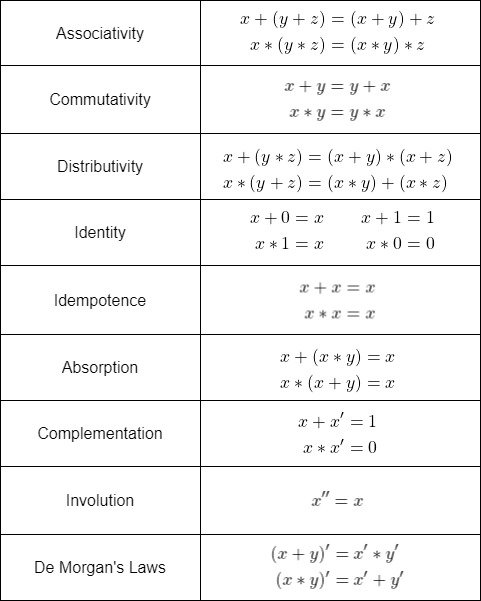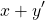# Mathematics - Discrete Mathematics - Boolean Algebra

in STEMGeekslast year[Image 1]

## Introduction

Hey it's a me again @drifter1!

Today we continue with Mathematics, and more specifically the branch of "Discrete Mathematics", in order to get into Boolean Algebra.

So, without further ado, let's get straight into it!

## Boolean Algebra

Boolean algebra is a branch of mathematics, which studies the algebra of 0 and 1, or false and true. It finds many applications in computer science, with logical circuits being the most direct application, but it's also useful in programming / coding in general.

## Boolean Operations

The three main boolean operations are:

• AND (conjunction)
• OR (disjunction)
• NOT (negation)

These are the same exact operations that were covered in the article about Propositional Logic.

### Notation

In Boolean algebra the AND and OR operations are commonly symbolized using multiplication and addition respectively, meaning that AND uses "*" or even nothing, and OR uses "+". Using the symbols ∧ and ∨ is of course also valid. Lastly, negation is usually represented by an apostrophe (') or an overline (-) instead of an tilde (~) in front of the respective boolean variable.

## Boolean Properties

Boolean operations satisfy the properties shown in the table below.Boolean algebra is basically a complemented, distributive lattice, with boolean operations AND (∧ or *), OR (∨ or +) and a unique complement or unary operation defined for each element. The elements are only two: 0 and 1.

## Boolean Expressions

Boolean expressions are expressions defined over boolean Algebra. Each element in such an expression is an boolean expression itself (i.e. 0 and 1 are boolean expressions on their own).

For example:is a valid boolean expressions with boolean variables x and y.

Such expressions are evaluated by assigning either 0 or 1 to each boolean variable, yielding a final result of 0 or 1.

### Equivalent Expressions

Of course, there can be multiple boolean expressions giving the same result for the same assignment of values. These so called equivalent expressions have the same truth table.

### Canonical Forms

It's common to write boolean expressions in either disjunctive or conjunctive normal form, and so basically as a sum of products (SOP) or a product of sums (POS). In a SOP, the product terms are known as min-terms (resulting in 1), whilst in a POS the sum terms are called max-terms (resulting in 0).

## RESOURCES:

### Images

Block diagrams and other visualizations were made using draw.io.

## Previous articles of the series

• Introduction → Discrete Mathematics, Why Discrete Math, Series Outline
• Sets → Set Theory, Sets (Representation, Common Notations, Cardinality, Types)
• Set Operations → Venn Diagrams, Set Operations, Properties and Laws
• Sets and Relations → Cartesian Product of Sets, Relation and Function Terminology (Domain, Co-Domain and Range, Types and Properties)
• Relation Closures → Relation Closures (Reflexive, Symmetric, Transitive), Full-On Example
• Equivalence Relations → Equivalence Relations (Properties, Equivalent Elements, Equivalence Classes, Partitions)
• Partial Order Relations and Sets → Partial Order Relations, POSET (Elements, Max-Min, Upper-Lower Bounds), Hasse Diagrams, Total Order Relations, Lattices
• Combinatorial Principles → Combinatorics, Basic Counting Principles (Additive, Multiplicative), Inclusion-Exclusion Principle (PIE)
• Combinations and Permutations → Factorial, Binomial Coefficient, Combination and Permutation (with / out repetition)
• Combinatorics Topics → Pigeonhole Principle, Pascal's Triangle and Binomial Theorem, Counting Derangements
• Propositions and Connectives → Propositional Logic, Propositions, Connectives (∧, ∨, →, ↔ and ¬)
• Implication and Equivalence Statements → Truth Tables, Implication, Equivalence, Propositional Algebra
• Proof Strategies (part 1) → Proofs, Direct Proof, Proof by Contrapositive, Proof by Contradiction
• Proof Strategies (part 2) → Proof by Cases, Proof by Counter-Example, Mathematical Induction
• Sequences and Recurrence Relations → Sequences (Terms, Definition, Arithmetic, Geometric), Recurrence Relations
• Probability → Probability Theory, Probability, Theorems, Example
• Conditional Probability → Conditional Probability, Law of Total Probability, Bayes' Theorem, Full-On Example
• Graphs → Graph Theory, Graphs (Vertices, Types, Handshake Lemma)
• Graphs 2 → Graph Representation (Adjacency Matrix and Lists), Graph Types and Properties (Isomorphic, Subgraphs, Bipartite, Regular, Planar)
• Paths and Circuits → Paths, Circuits, Euler, Hamilton
• Trees → Trees (Rooted, General and Binary), Tree Traversal, Spanning Trees
• Common Graph Problems → Shortest Path Problem, Graph Connectivity, Travelling Salesman Problem, Minimum Spanning Tree, Maximum Network Flow, Graph Coloring
• Binary Operations → Binary Operations (n-ary, Table Representation), Properties
• Groups → Groups (Properties, Theorems, Finite and Infinite, Abelian, Cyclic, Product, Homo-, Iso- and Auto-morphism)
• Group-like Structures (part 1) → Subgroups, Semigroups, Monoids
• Group-like Structures (part 2) → Magma, Quasigroup, Groupoid
• Rings → Rings (Axioms, Commutative and Non-Commutative, Semirings, Subrings, Rng)
• Fields → Fields (Axioms, Subfields, Finite Fields, Field Extension)

## Final words | Next up

And this is actually it for today's post!

Not sure about the next article's topic yet.

See ya!Keep on drifting!

Posted with STEMGeeks

Sort:

!1UP#### You have received a 1UP from @luizeba!

The following @oneup-cartel family members will soon upvote your post:
`@stem-curator`
And they will bring !PIZZA 🍕